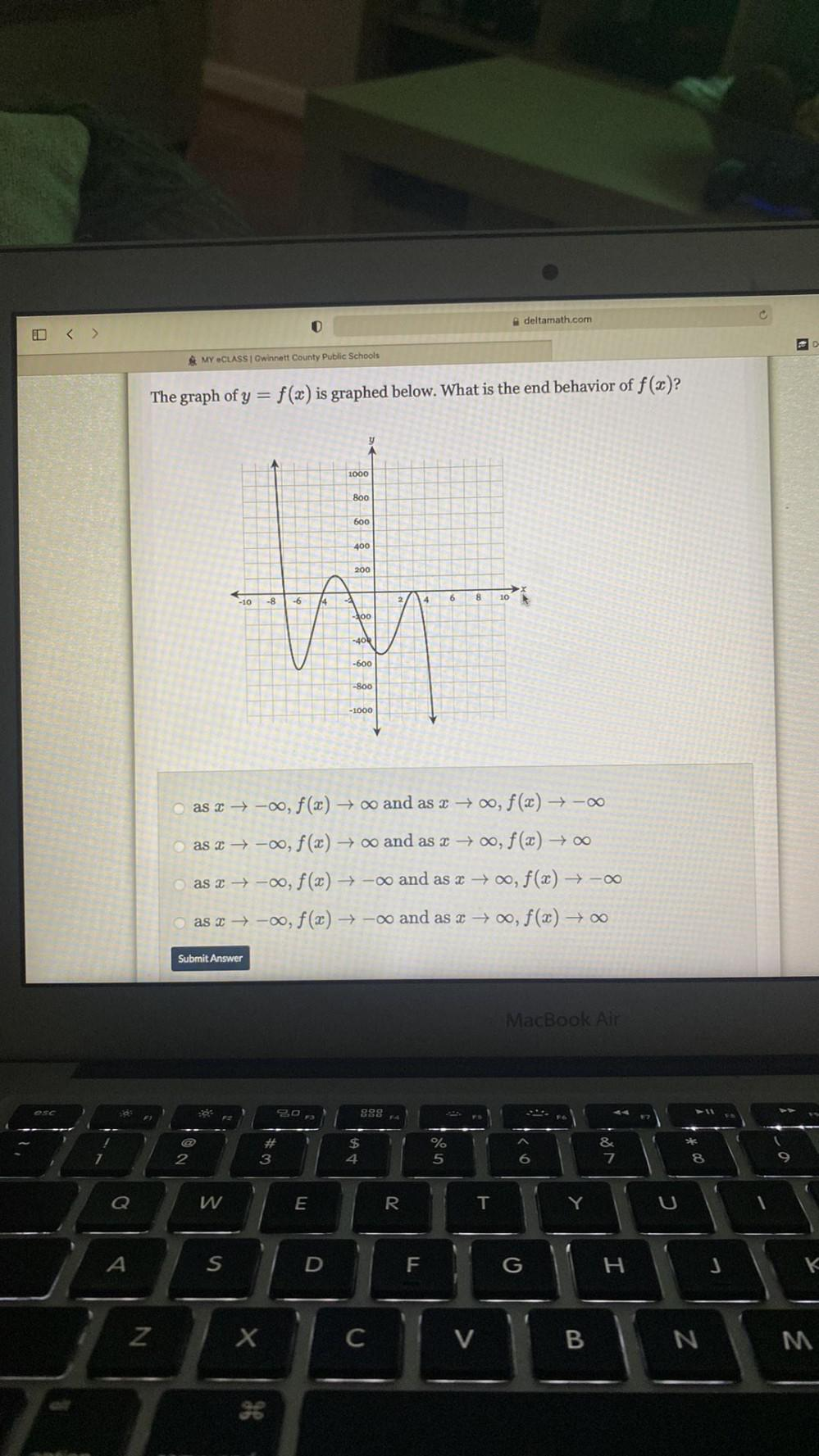Question:

# deltarnath.com 0 D & MY CLASS Gwinnett County Public Schools The graph of y = f(x) is graphed below. What is the end behavior ofdeltarnath.com 0 D & MY CLASS Gwinnett County Public Schools The graph of y = f(x) is graphed below. What is the end behavior of f(x)? 1000 800 600 400 200 6 8 10 -10 -8 14 200 -404 -600 -800 -1 -1000 as 2 →-0, f(x) and as x +00, f(x) +- as 2 → -00, f(x) and as x → 00, f(x) +0 as -o, f(x) + -oo and as x → , f(x) + - as 27 -00, f(x) - and as x +00, f(x) +0 Submit Answer MacBook Air Ó: # 3 \$ 4 % 5 KO & 7 2 8 o Q W w E R T Y A S F G H Z X C V B N M 36